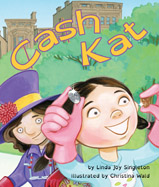Alignment to Standards for MN1 1.2.2.1 Represent real-world situations involving addition and subtraction basic facts, using objects and number sentences.
1 1.2.2.4 Use addition or subtraction basic facts to represent a given problem situation using a number sentence.
1 1.3.2.3 Identify pennies, nickels and dimes; find the value of a group of these coins, up to one dollar.
2 2.3.3.2 Identify pennies, nickels, dimes and quarters. Find the value of a group of coins and determine combinations of coins that equal a given amount.
3 3.1.3.1 Read and write fractions with words and symbols. Recognize that fractions can be used to represent parts of a whole, parts of a set, points on a number line, or distances on a number line.
3 3.1.3.2 Understand that the size of a fractional part is relative to the size of the whole.
4 4.1.2.4 Read and write decimals with words and symbols; use place value to describe decimals in terms of thousands, hundreds, tens, ones, tenths, hundredths and thousandths.

Back to Standards Page